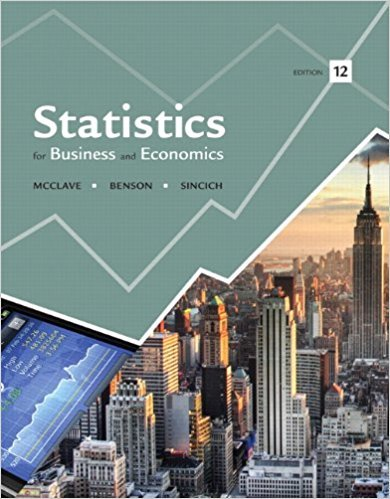×
Get Full Access to Statistics For Business And Economics - 12 Edition - Chapter 7 - Problem 145se
Get Full Access to Statistics For Business And Economics - 12 Edition - Chapter 7 - Problem 145se

×

# The Hot Tamale caper. “Hot Tamales” are chewy, cinnamonISBN: 9780321826237 51

## Solution for problem 145SE Chapter 7

Statistics for Business and Economics | 12th Edition

• Textbook Solutions
• 2901 Step-by-step solutions solved by professors and subject experts
• Get 24/7 help from StudySoup virtual teaching assistantsStatistics for Business and Economics | 12th Edition

4 5 1 272 Reviews
15
5
Problem 145SE

Problem 145SE

The Hot Tamale caper. “Hot Tamales” are chewy, cinnamon flavored candies. A bulk vending machine is known to dispense, on average, 15 Hot Tamales per bag. Chance (Fall 2000) published an article on a classroom project in which students were required to purchase bags of Hot Tamales from the machine and count the number of candies per bag. One student group claimed it purchased five bags that had the following candy counts: 25, 23, 21, 21, and 20. There was some question as to whether the students had fabricated the data. Use a hypothesis test to gain insight into whether or not the data collected by the students were fabricated. Use a level of significance that gives the benefit of the doubt to the students.

Step-by-Step Solution:

Solution :

Step 1 of 1:

Given 1 student group claimed it purchased 5 bags.

Then the table is given below.

 Candy 25 23 21 21 20

We are using Minitab to find the mean and standard deviation.

Now we have to follow the steps.

1). Enter the data in Minitab.

2). Select stat > Basic stat > Display Descriptive Statistics.

3). In variable enter the plants.

Then click ok.

The table is given below.When it is true to favour the students we do not reject the null hypothesis.

Here the sample comes from a normal distribution.

Now we have to determine if the mean number of candies exceeds 15.

Then the null and alternative hypothesis areagainestThen the test statistic formula is

z =z =We know that the mean, n=5,, and standard deviation=2.

z =z =z = 7.82

Therefore, the value of the test statistic z is 7.82.

We know that the level of significancein the upper tail of the z-distribution.

Then from table II, Appendix Dis.So the rejection region is z > 3.08.

Hence  z = 7.83>3.08.

Now we rejected the null hypothesis.

Therefore, we conclude that there is sufficient evidence to indicate the mean number of candies exceeds 15 at the level of significance is 0.001.

Step 2 of 1

##### ISBN: 9780321826237

Unlock Textbook Solution